Processing ......FreeComputerBooks.com Links to Free Computer, Mathematics, Technical Books all over the World

Combinatorics and Game Theory
Related Book Categories:
 Game TheoryAlgebra and Abstract Algebra Algorithms and Data Structures Discrete and Finite Mathematics
•Combinatorics Through Guided Discovery (Kenneth P. Bogart)

This book is an introduction to combinatorial mathematics, also known as combinatorics. The book focuses especially but not exclusively on the part of combinatorics that mathematicians refer to as 'counting'.

•Applied Combinatorics (Mitchel T. Keller, et al)

This is a text with more than enough material for a one-semester introduction to combinatorics. The original target audience was primarily computer science majors, but the topics included make it suitable for a variety of different students.

•Analytic Combinatorics (Philippe Flajolet, et al)

The definitive treatment of analytic combinatorics. This self-contained text covers the mathematics underlying the analysis of discrete structures, with thorough treatment of a large number of applications.

•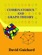An Introduction to Combinatorics and Graph Theory

This book walks the reader through the classic parts of Combinatorics and graph theory, while also discussing some recent progress in the area: on the one hand, providing material that will help students learn the basic techniques.

•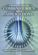Foundations of Combinatorics with Applications (Edward Bender)

This introduction to combinatorics, the foundation of the interaction between computer science and mathematics, is suitable for upper-level undergraduates and graduate students in engineering, science, and mathematics.

•Lists, Decisions and Graphs - With an Introduction to Probability

In this book, four basic areas of discrete mathematics are presented: Counting and Listing (Unit CL), Functions (Unit Fn), Decision Trees and Recursion (Unit DT), and Basic Concepts in Graph Theory (Unit GT).

•Magic Squares and Cubes (William Symes Andrews)

This book cover topics such as magic squares, magic cubes, the Franklin squares, magics and Pythagorean numbers, the theory of reversions, magic circles, spheres, and stars, and magic octahedroids, among other things.

•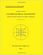Combinatorial Geometry with Application to Field Theory

Topics covered in this book include fundamental of combinatorics, algebraic combinatorics, topology with Smarandache geometry, combinatorial differential geometry, combinatorial Riemannian submanifolds, Lie multi-groups, etc.

•Notes on Convex sets, Polytopes, Polyhedra, Combinatorial Topology

This book may be viewed as a tutorial and a set of notes on convex sets, polytopes, polyhedra, combinatorial topology, Voronoi Diagrams and Delaunay Triangulations.

•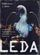LEDA: A Platform for Combinatorial and Geometric Computing

LEDA is a library of efficient data types and algorithms and a platform for combinatorial and geometric computing. This book, written by the main authors of LEDA, is the definitive account of how the system operates and how it can be used.

•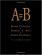A=B, by Marko Petkovsek, Herbert S. Wilf, et al

This book is of interest to mathematicians and computer scientists working in finite mathematics and combinatorics. It presents a breakthrough method for analyzing complex summations.

•Algebraic Combinatorics on Words (M. Lothaire)

This book is both a comprehensive introduction to the subject and a valuable reference source for researchers.

•Applied Combinatorics on Words (M. Lothaire)

The aim of this book is to present a unified treatment of some of the major fields of applications of combinatorics.

•Combinatorial Algorithms for Computers and Calculators (H. S. Wilf)

On one level, this is a collection of subroutines, in FORTRAN, for the solution of combinatorial problems.

•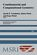Combinatorial and Computational Geometry (Jacob E. Goodman)

It includes surveys and research articles exploring geometric arrangements, polytopes, packing, covering, discrete convexity, geometric algorithms and their complexity, and the combinatorial complexity of geometric objects, particularly in low dimension.

•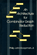Architecture for Combinator Graph Reduction (Philip J. Koopman)

The results of cache-simulation experiments with an abstract machine for reducing combinator graphs are presented.

•Knapsack Problems: Algorithms and Computer Implementations

The text fully develops an algorithmic approach to Knapsack Problems without losing mathematical rigor.

•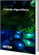Greedy Algorithms (Witold Bednorz)

This book covers fundamental, theoretical topics as well as advanced, practical applications of Greedy Algorithms.

•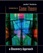Introduction to Game Theory: a Discovery Approach

This book includes an exploration of the ideas of Game Theory through the rich context of popular culture. It contains sections on applications of the concepts to popular culture. It suggests films, television shows, and novels with themes from game theory.

•Game Theory: An Open Access Textbook (Giacomo Bonanno)

This book is an introduction to Game Theory. Accessible to anybody with minimum knowledge of mathematics and no prior knowledge of game theory, yet it is also rigorous and includes several proofs.

•Game Theory Relaunched (Hardy Hanappi)

The game is on. Do you know how to play? Game Theory sets out to explore what can be said about making decisions which go beyond accepting the rules of a game. New simulation tools and network analysis have made game theory omnipresent these days.

•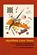Algorithmic Game Theory (Noam Nisan, et al)

This book covers many of the hottest area of useful new Game Theory research, introducing deep new problems, techniques, and perspectives that demand the attention of economists as well as computer scientists.

•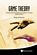Game Theory: A Nontechnical Introduction

Striking an appropriate balance of mathematical and analytical rigor, it teaches game theory by examples - serves as an introduction to game theory for students with no prior game theory knowledge, or with limited background in economics and mathematics.

•Logic and the Foundations of Game and Decision Theory

This book collects together revised papersoriginally presented at the 7th Conference onLogic and the Foundations of Game and DecisionTheory (LOFT). LOFT is a key venue forpresenting research at the intersection of logic,economics and computer science.

•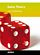Game Theory (Qiming Huang)

This book provides a powerful mathematical framework that can accommodate the preferences and requirements of various stakeholders in a given process as regards the outcome of the process.

•Games of No Chance (Richard Nowakowski, editor)

This book deals with combinatorial games, that is, games not involving chance or hidden information.

•More Games of No Chance (Richard J. Nowakowski)

This fascinating collection of articles by some of the top names in the field is a state-of-the-art look at combinatorial games.

•Games of No Chance 3 (Michael H. Albert, et al)

This fascinating look at combinatorial games, that is, games not involving chance or hidden information, offers updates on standard games such as Go and Hex, on impartial games such as Chomp and Wythoff's Nim, etc.

•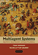Multiagent Systems: Algorithmic, Game-Theoretic, and Logic, etc.

This comprehensive introduction to a burgeoning field is written from a computer science perspective, while bringing together ideas from operations research, game theory, economics, logic, and even philosophy and linguistics.

•A Beautiful Math: John Nash, Game Theory, and a Code of Nature

Today neuroscientists peer into game players brains, anthropologists play games with people from primitive cultures, biologists use games to explain the evolution of human language, and mathematicians exploit games to better understand social networks.

•Games, Fixed Points and Mathematical Economics (C. Ewald)

This book gives the reader access to the mathematical techniques involved and goes on to apply fixed point theorems to proving the existence of equilibria for economics and for co-operative and noncooperative games.

•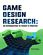Game Design Research (Petri Lankoski, et al)

Design research is an active academic field covering disciplines such as architecture, graphic, product, service, interaction, and systems design. The book demonstrates different approaches to design research in game design research.

Book Categories
 :All CategoriesTop BooksRecent BooksMiscellaneous BooksComputer LanguagesComputer ScienceData Science/DatabasesElectrical EngineeringJava and Java EE (J2EE)Linux and UnixMathematicsMicrosoft and .NETMobile ComputingNetworking and CommunicationsSoftware EngineeringSpecial TopicsWeb Programming
Other Categories